# CBSE Class 10 Science Sample Paper Solved 2021 Term 1 Set A

Read and download PDF of CBSE Class 10 Science Sample Paper Solved 2021 Term 1 Set A designed as per the latest curriculum and examination pattern for Class 10 issued by CBSE, NCERT and KVS. The latest Class 10 Science Sample Papers have been provided with solutions so that the students can solve these practice papers and then compare their answers. This will help them to identify mistakes and improvement areas in Science Standard 10 which they need to study more to get better marks in Grade 10 exams. After solving these guess papers also refer to solved Class 10 Science Question Papers available on our website to build strong understanding of the subject

## Sample Paper for Class 10 Science 2021 Pdf

Students can refer to the below Class 10 Science Sample Paper designed to help students understand the pattern of questions that will be asked in Grade 10 exams. Please download CBSE Class 10 Science Sample Paper Solved 2021 Term 1 Set A

### Science Class 10 Sample Paper 2021

Section A
Section – A consists of 24 questions. Attempt any 20 questions from this section.
The first attempted 20 questions would be evaluated.

Question 1. Translate the following statement into the chemical equation and choose the correct option “Hydrogen gas combines with nitrogen to form ammonia.”
(a) 3H2(g) + N2(g) →2NH3(g)
(b) H2(g) + N2(g) NH2(g) +
(c) 2H(g) + N2(g) 2NH3
(d) None of these

Question 2. In an experiment to study the properties of acetic acid, a student takes about 2 ml of acetic acid in a dry test tube. He adds about 2 ml of water to it and shakes the test tube well. What will he observe ?
(a) The acetic acid dissolves readily in water.
(b) The solution becomes light orange.
(c) Water floats over the surface of acetic acid.
(d) Acetic acid floats over the surface of water.

Question 3. When a metal X is treated with cold water, it gives a base Y with molecular formula XOH (Molecular mass = 40) and liberates a gas Z which easily catches fire. Here X, Y and Z are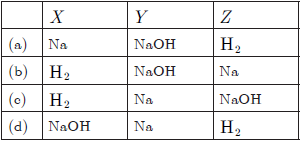Question 4. In the reaction Br2 + 2I →2Br- + I2  , the oxidising agent is:
(a) Br2 (b) I
(c) Br− (d) I2

Question 5. Which of the following are responsible for causing acid-rain?
(a) Oxides of sulphur and carbon dioxide
(b) Oxides of nitrogen and carbon monoxide
(c) Hydrogen sulphide and carbon dioxide
(d) Oxides of sulphur and nitrogen

Question 6. CuO + H2 →H2O + Cu , reaction is an example of:
(a) redox reaction
(b) synthesis reaction
(c) neutralisation
(d) analysis reaction

Question 7. 3MnO2 + 4Al → 3Mn + 2Al2O3
The oxidising agent in the above reaction is:
(a) MnO2 (b) Al
(c) Mn (d) Al2O3

Question 8. Arrange the following acids on their basicity in the table:
A = HCl, B = HNO3, C = H3PO4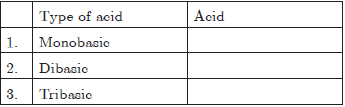(a) 1-A, 2-B, 3-C
(b) 1-C, 2-B, 3-A
(c) 1-A, 2-C, 3-B
(d) 1-B, 2-C, 3-A

Question 9. Which of following in not a natural indicator?
(a) Red cabbage (b) China rose
(c) Turmeric (d) Onion

Question 10. A dilute solution of sodium carbonate was added to two test tubes - one containing dil HCl (A) and the other containing dilute NaOH(B). Which of the following was the correct observation?
(a) A brown coloured gas liberated in test tube A.
(b) A brown coloured gas liberated in test tube B.
(c) A colourless gas liberated in test tube A.
(d) A colourless gas liberated in test tube B.

Question 11. Name the term which means ‘taking in of air rich in oxygen into the body’.
(a) Exhalation (b) Inhalation
(c) Breathing (d) Respiration

Question 12. Identify the secretion inside the stomach which helps to protect the inner lining of stomach from the action of acid
(a) Mucus (b) Hydrochloric acid
(c) Digestive juices (d) Enzymes

Question 13. The process by which autotrophs take in substances from the outside and convert them into stored forms of energy is known as
(a) Photosynthesis (b) Respiration
(c) Molecular breakup (d) Cell diffusion

Question 14. Oxygenated blood is pumped from heart to rest of the body via ?
(a) Aorta (b) Pulmonary
(c) Pulmonary vein (d) Vena cava

Question 15. Which of the following organ is not involved in excretion?
(a) Lungs (b) Liver
(c) Stomach (d) Skin

Question 16. The process of movement of solvent particles from region of less solute concentration to region of high solute concentration through semi permeable membrane is called ...........
(a) Diffusion (b) Osmosis
(c) Transpiration (d) Translocation

Question 17. A ray of light falls normally on the surface of a transparent glass slab. The angle of emergence is-
(a) 0° (b) 90°
(c) 45° (d) 70°

Question 18. An object is placed 20 cm from the concave mirror of focal length 10 cm, then image is formed at
(a) centre of curvature of mirror
(b) behind the mirror
(c) between the mirror and focus
(d) at focus

Question 19. A 6 cm tall object is placed perpendicular to the principal axis of a convex lens of focal length 15 cm. The distance of the object from the lens is 10 cm. The position, of the image is-
(a) 20 cm (b) 30 cm
(c) −30 cm (d) 50 cm

Question 20. A convex lens has a focal length of 10 cm. At what distance from the lens should the object be placed so that it forms a real and inverted image 20 cm away from the lens?
(a) −20 cm (b) −40 cm
(c) −60 cm (d) −80 cm

Question 21. As light travels from a rarer to a denser medium it will have
(a) increased velocity
(b) decreased velocity
(c) decreased wavelength
(d) both (b) and (c)

Question 22. The focal length of a spherical mirror is:
(a) Maximum for red light
(b) Maximum for blue light
(c) Maximum for white light
(d) Same for all lights

Question 23. Which of the following defines the center of curvature of a curved mirror?
(a) The center of the follow glass sphere of which the curved mirror was (previously) a part
(b) The geometric centre of the curved mirror
(c) The radius of the hollow glass sphere of which the curved mirror was (previously) a part
(d) None of these

Question 24. How many times does a ray of light bend on passing through a prism?
(a) Once (b) Twice
(c) Thrice (d) Four times

Section B
Section - B consists of 24 questions (Sl. No.25 to 48). Attempt any 20 questions from this section.
The first attempted 20 questions would be evaluated.

Question 25. The hydrogen ion concentration of solution is 0.001 M. The pH of solution is?
(a) 3 (b) 2
(c) 1 (d) 1.5

Question 26. To a sample of turmeric adulterated with metanil yellow, concentrated hydrochloric acid was added. The colour of the reaction mixture
(a) became green (b) became blue
(c) remained the same (d) disappeared

Question 27. The state in which NaCl is bad conductor of electricity is _________ but, the state in which NaCl is good conductor of electricity is __________.
(a) solid, vapour (b) solid, molten
(c) vapour, molten (d) molten, solid

Question 28. A metal which reacts with cold water vigorously producing hydrogen is:
(a) sodium (b) mercury
(c) tungsten (d) zinc

Question 29. When NaOH and HCl are mixed in equal molar quantities, the result is
(a) the formation of salt +H2O
(b) the formation of salt +H2(g)
(c) the formation of salt +O2(g)
(d) All above are correct

Question 30. Which of the following metals on reacting with sodium hydroxide solution produce hydrogen gas?
1. Cu
2. Al
3. Fe
4. Zn
(a) 2 and 3 (b) 2 and 4
(c) 1 and 4 (d) 2 only

Question 31. Assertion : Non-metallic oxides are acidic is nature.
Reason : Non-metallic oxides react with base to form  salt and water just like an acid reacts with a base.
(a) Both Assertion and Reason are true and Reason is the correct explanation of the Assertion.
(b) Both Assertion and Reason are true but Reason is not the correct explanation of the Assertion.
(c) Assertion is true but the Reason is false.
(d) Both Assertion and Reason are false.

Question 32. Assertion : Equation
C(s) + O2(g) → CO2(g)  is an example of combination reaction.
Reason : In the given above equation, carbon and oxygen react to give carbon dioxide.
(a) Both Assertion and Reason are True and Reason is the correct explanation of the Assertion.
(b) Both Assertion and Reason are True but Reason is not the Correct explanation of the Assertion.
(c) Assertion is True but the Reason is False.
(d) Both Assertion and Reason are False.

Question 33. Assertion : Carbon monoxide is injurious to the health of the individual.
Reason : Carbon monoxide has very strong affinity for the blood.
(a) Both Assertion and Reason are true and Reason is the correct explanation of Assertion.
(b) Both Assertion and Reason are true but Reason is not the correct explanation of Assertion.
(c) Assertion is true but Reason is false.
(d) Both Assertion and Reason are false.

Question 34. Assertion : Sunlight reaches us without dispersion in the form of white light and not as its components.
Reason : Dispersion takes place due to variation of refractive index for different wavelength but in vacuum the speed of light is independent of wavelength and hence vacuum is a non-dispersive medium.
(a) Both Assertion and Reason are true and Reason is the correct explanation of Assertion.
(b) Both Assertion and Reason are true but Reason is not the correct explanation of Assertion.
(c) Assertion is true but Reason is false.
(d) Assertion is false but Reason is true.

Question 35. Which of the following substances will not give carbon dioxide on treatment with dilute acid?
(a) Marble (b) Limestone
(c) Baking soda (d) Lime

Question 36. The science teacher ordered to Mohit to do the following acativity with magnesium ribbon:
1. Clean a magnesium ribbon about 3-4 cm long by rubbing it with sandpaper.
2. Hold it with a pair of tongs. Burn it using a spirit lamp or burner and collect the ash so formed in a watch-glass as shown in Figure. Burn the magnesium ribbon keeping it away as far as possible from your eyes.
Which of the following reaction take place here?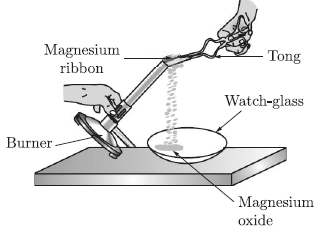(a) 2Mg + O2 →2MgO
(b) 2Mg + H2 →2MgH
(c) 2Mg + N2 →2MgN
(d) 2Mg + He2 →2MgHe

Question 37. The thick stem of trees respire through
(a) Trachea (b) Stomata
(c) Lenticel (d) Gills

Question 38. The excretory unit of the human excretory system is known as?
(a) Nephridia (b) Neuron
(c) Nephron (d) kidneys

Question 39. The path of a ray of light coming from air passing through a rectangular glass slab traced by four students are shown in figure. Which one of them is correct?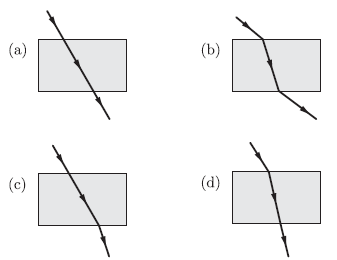Question 40. Which of the following correctly represents graphical relation between angle of incidence (i) and angle of reflection (r)?Question 41. Which process is shown by the following picture?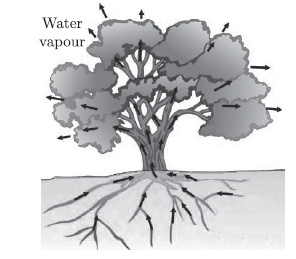(a) Movement of food during photosynthesis in a tree
(b) Movement of water during transpiration in a tree
(c) Movement of minerals during in a tree
(d) Movement of carbon dioxide during in a tree

Question 42. An artificial kidney is a device to remove nitrogenous waste products from the blood through-
(a) Diaphragm (b) Dialysis
(c) ECG (d) Electrolysis

Question 43. In which of the following, the image of an object placed at infinity will be highly diminished and point sized?
(a) Concave mirror only
(b) Convex mirror only
(c) Convex lens only
(d) Concave mirror, convex mirror, concave lens and convex lens

Question 44. If the central portion of a convex lens is wrapped in black paper as shown in the figure(a) No image will be formed by the remaining portion of the lens
(b) The full image will be formed but it will be less bright
(c) The central portion of the image will be missing
(d) There will be two images each produced by one of the exposed portions of the lens

Question 45. An object is kept in front of a concave mirror of focal length 20 cm. The image is three times the size of the object. The possible distances of the object from the mirror is-
(a) −80/3 (b) −40/3
(c) −50/3 (d) −10/3

Question 46. A convex lens of focal length 25 cm and a concave lens of focal length 10 cm are placed in close contact with each other. The power of this combination is-
(a) 2 D (b) 6 D
(c) −6 D (d) 9 D

Question 47. A convex lens of focal length 20 cm is placed in contact with a concave lens of focal length 10 cm. The power of the combination is-
(a) 10 dioptre (b) −10 dioptre
(c) −5 dioptre (d) 5 dioptre

Question 48. Which of the following metals gives hydrogen with dil. HNO3?
(a) Al (b) Mg
(c) Az → (d) Sn

Section C

Section- C consists of three Cases followed by questions. There are a total of 12 questions in this section. Attempt any 10 questions from this section.
The first attempted 10 questions would be evaluated.

Case Based Questions: (49-52)
The pH of a solution is a measure of its hydrogen ion (H+) concentration. It is measured generally using pH scale. The values on pH scale ranges from 0 to 14.
A pH of 1 is very acidic and corresponds to a high concentration of H+ ions. A pH of 14 is very basic and corresponds to a low concentration of H+ ions.
The pH of a neutral solution is 7. The table given below shows the pH and H+ ion concentration of some common aqueous solutions. The leftmost column shows the number of moles of H+ ions in 1 mole of liquid.
The pH and Hydrogen ion (H+) Concentration of Some SolutionsQuestion 49. How is the hydrogen ion concentration and pH related to each other?
(a) They are inversely proportional
(b) They are directly proportional
(c) They are equal
(d) They have no relation

Question 50. Among the given solutions in the above table, the most basic in nature is
(a) pure water (b) oven cleaner
(c) household bleach (d) gastric juice

Question 51. The acid having highest hydrogen ion concentration is one with
(a) pH = 2.5 (b) pH = 1.8
(c) pH = 7 (d) pH = 10

Question 52. Which of the following acids is used in making of vinegar?
(a) Nitric acid
(b) Sulphuric acid
(c) Formic acid
(d) Acetic acid

Case Based Questions: (53-56)

The excretory system is a passive biological system that removes excess, unnecessary materials from the body fluids of an organism, so as to help maintain internal chemical homeostasis and prevent damage to the body.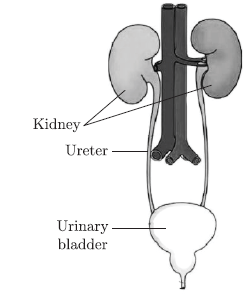Question 53. Which among the following is the storage organ for urine?
(a) Urter (b) Kidney

Question 54. The main function of ureter is
(a) contain urine till is released out
(b) carry urine from kidney to urinary bladder
(c) guard the urethra
(d) passage through which urine is excreted out of the body

Question 55. The diagram shows a kidney and its associated vessels.Which structure have the most and least urea concentration?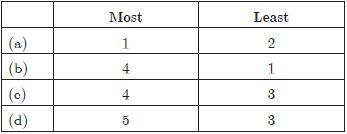Question 56. If Henle’s loop were absent from mammalian nephron, which one of the following to be expected?
(a) There will be no urine formation
(b) There will be hardly any change in the quality and quantity of urine formed
(c) The urine will be more concentrated
(d) The urine will be more dilute

Case Based Questions: (57-60)
Refer to the path of rays of light through a glass prism. Now answer the following questions.Question 57. A glass prism has
(a) six rectangular surfaces
(b) four rectangular surfaces
(c) two triangular bases and three rectangular surfaces
(d) none of the above.

Question 58. In passing through a glass prism, a ray of light undergoes how many refractions?
(a) One (b) Two
(c) Three (d) Four